# Percentage Change Formula In ExcelArticle byExcelMojo Team## What Is Percentage Change Excel Formula?

The percentage change formula in Excel determines the percentage variance between the two specified values, original and new. It subtracts the original value from the new value and divides the difference by the original value. Users can use the formula to assess the percentage difference for an entity between two periods. For example, we can assess the total number of cars manufactured in the last and current years.

For example, the below table contains the 2017-21 estimated and actual US population statistics.

Suppose the requirement is to determine the yearly percentage variance in the US population and display the results in cell range D2:D6. Then, applying the percentage change formula cells in the range D2:D6 can fetch us the required data.

Assume the target cells have the data format set as Percentage in HomeNumber Format (highlighted in the above image).

In the above percentage change formula in Excel example, each target cell expression finds the difference between the actual and estimated population values in each row. And then, it divides the difference value by the estimated population to return the corresponding year’s percentage change in the US population.

###### Key Takeaways
• The percentage change formula in Excel computes the difference between an entity’s new and old values and then divides the variance by the old value to give its percent change.
• Users can utilize the percentage change formula to determine the duration-wise percentage variance for an entity, such as for a firm’s revenue in the two specified years.
• Using the percentage change formula, you can calculate the value after percent increase/decrease, the absolute value of a percent change, and the discount percent. The formula also helps determine the percentage change, even when the input values include zeros and negative values.

### Excel Formula For Percentage Increase/Decrease

An Excel formula for a percentage increase or decrease is a type of percentage change formula in Excel, and its equation is:

Percentage Increase/Decrease = (New_Value – Initial_Value) / (Initial_Value)

Or

Percentage Increase/Decrease = New_Value / Initial_Value – 1

For example, the below table contains a list of athletes and their lap time data for two races.

Suppose we must determine the percentage change in the lap time, based on the two races’ lap times, for each athlete and display the results in columns E and F. Assume the data format of columns E and F is Percentage (highlighted in the above image).

Then, applying the first formula in column E and the second in column F will give the required data, with both formulas resulting in the same outcome.

• Step 1: Select the target cell E3, enter the below formula, and press Enter.

=(D3-C3)/C3

• Step 2: Select the target cell F3, enter the below formula, and press Enter.

=D3/C3-1

• Step 3: Select cells in the range E3:F3, and using the fill handle in Excel, implement the above formulas in the cell range E4:F12.

The above percentage change formula in Excel example shows the outcomes of the percent increase/decrease formulas in columns E and F are the same.

Here, the Race 1 lap time is the Initial_Value, and the Race 2 lap time is the New_Value. So, in rows where the Race 1 lap time is more than the Race 2 lap time. Therefore, the percentage increase/decrease formulas return a negative value. Otherwise, their outcome is positive.

On the other hand, in row 5, the lap times in both races for Athlete 3 are the same. And hence, their difference is 0, which results in a 0% percentage variance. It indicates that there is no percentage increase or decrease in Athlete 3’s lap times.

### Calculating Value After Percent Increase Or Decrease

If we require to compute the value of an entity after a percent increase or decrease, the percentage change formula in Excel we need to use is:

Value After Percent Increase or Decrease = Initial_Value * (1 + Percent_Change)

For example, the following table contains a list of products, their prices in 2021, and the percentage variations in 2022, compared to 2021 figures.

Suppose we must determine the updated price of each product for 2022 based on their 2021 prices and percentage variations in prices in 2022 and display the results in column E.

Assume column E data format is Currency in HomeNumber Format (as highlighted in the above image).

Then, applying the above-mentioned formula in the target cells will fetch us the required data.

• Step 1: Select the target cell E3, enter the below formula, and press Enter.

=C3*(1+D3)

• Step 2: Use the fill handle to enter the above formula in cell range E4:E7.

Let us consider the cell E7 expression to understand how the formula works.

The formula takes Product 5’s 2021 price as the Initial_Value and its 2022 percentage variation in the price as the Percent_Change.

It first adds one to the 2022 percentage variation in price, -25%, to determine the total percentage, 75%. And then, it multiplies the computed total percentage of 75% with the 2021 price, \$250, to give Product 5’s updated price in 2022 as \$187.50.

As the percentage variation is negative, the new price of Product 5 in 2022 will be less than the 2021 price.

On the other hand, suppose the price variation is the same for all products, say, 8%. Then, the above-mentioned formula becomes:

Value After Percent Increase or Decrease = Initial_Value * (1 + 8%)

However, chances are we might ignore the ‘%’ symbol. Hence, the suggestion is to enter the price variation value in a cell and use the absolute cell reference to the value in the above formula. It will help avoid the situation of the percentage change formula in Excel not working.

For example, we shall modify the source table to explain the above scenario.

Cell C9 contains the percentage variation in price in 2022 for all the products. And suppose we require to determine the new price in 2022 for each product based on the corresponding 2021 price and the percentage variation in price in 2022.

Assume the target cells are in the range C11:C15, with their data format set in HomeNumber Format as Currency.

Then, using the above formula in the target cells will help us achieve the required data.

• Step 1: Select the target cell C11, enter the below formula, and press Enter.

=C3*(1+\$C\$9)

• Step 2: Enter the formula in cell range C12:C15 using the fill handle.

The percentage variation in price in 2022 is positive. So, the new prices in 2022 for all the products stand higher than their 2021 prices.

On the other hand, using the absolute Excel reference to cell C9 ensures each target cell uses the same percentage value, 8%. And thus, we also avoid the condition of the percentage change formula in Excel not working while copying the formula in all the target cells.

### Percent Change Absolute Value

We observed in the previous sections that the percentage change formula in Excel returns a positive or negative percent change. However, the requirement might be to determine the absolute value of the percentage change. In such a scenario, we can use the percentage change formulas within the ABS() excel function as shown below:

Percent Change Absolute Value = ABS ((New_value – Old_Value) / Old_Value)

Or

Percent Change Absolute Value = ABS (New_Value / Old_Value – 1)

For example, the following table shows the monthly estimated and actual precipitation and the percentage change in precipitation using the usual percentage change formula.

Suppose the requirement is to determine the absolute value of the percent change in precipitation for each month and display the result in column F. Then, applying the following month-over-month percentage change formula in Excel cell range F3:F8 will help us achieve the required outcome.

Assume columns E and F cells have the data format set as Percentage in HomeNumber Format.

• Step 1: Select the target cell F3, enter the following formula, and press Enter.

=ABS((D3-C3)/C3)

• Step 2: Using the fill handle, update the above formula in cell range F4:F8.

Alternatively, we can apply the second formula the same way explained for the first formula (steps 1 and 2) to get the above result.

The percentage change formulas in column E return positive and negative values. The percent change is negative when the actual precipitation (New_Value) is less than the estimated precipitation (Old_Value).

However, the output of each month-over-month percentage change formula in Excel cell range F3:F8 is only positive. It is because of providing the percentage change formula in the ABS(), which returns the absolute value of the percent change.

### Calculating Discount Percentage

The percentage change formula in Excel for calculating the discount percentage on a commodity is:

Discount % = ABS ((Discounted_Price – List_Price) / List_Price))

Or

Discount % = ABS (Discounted_Price / List_Price)

For example, the following table shows an item list, their list, and discounted prices.

Suppose we must determine the discount % on each item and display the output in column E. Then, applying the above discount % formulas in the target cells will fetch us the required data.

Assume the target cells have the data format set as Percentage in HomeNumber Format.

• Step 1: Select the target cell E3, enter the discount % formula, and press Enter.

=ABS((D3-C3)/C3)

• Step 2: Using the fill handle, enter the above formula in cell range E4:E7.

Alternatively, we can apply the second formula the same way explained for the first formula (steps 1 and 2) to get the above result.

The discounted price is the amount after deducting the discount % from the list price. And thus, it will be less than the list price. And as the above formulas subtract the list price from the discounted price, the percentage formula output will be negative. But typically, a discount % is a positive value. And hence, we apply the discount % formula inside the ABS() to get the absolute value of the percentage.

### Percentage Change In Excel With Zero

We might face scenarios where an entity’s value changes from 0 to a new value or from an initial value to 0.

In such cases, we can get an incorrect answer.

Thus, the suggestion is to apply the appropriate percentage change formula in Excel to maintain the solution as 100%.

For example, the first scenario in the below table shows a value change from 0 to 100. And in the other, the value changes from 100 to 0.

And the table also shows the percentage change in each scenario when we apply the percentage change formula as explained in the previous sections.

As the denominator will be 0 in the percent change formula in the first scenario, the formula output is the #DIV/0! error. And as the final value is less than the initial value in the second scenario, the percent change formula output, in this case, will be negative.

Suppose the requirement is to show the ideal solution of percent change for the two scenarios in cell range F3:F4. Then, assuming the target cells’ data format is Percentage, applying the following formulas will help us achieve the desired outcome.

• Step 1: Select the target cell F3, enter the formula shown in the Formula Bar, and press Enter.
• Step 2: Select the target cell F4, enter the formula shown in the Formula Bar, and press Enter.

The ABS() returns the absolute value of the percentage change formula output.

Thus, keeping the denominator the same as the specified non-zero value will result in the percentage change formula returning a value of 100%.

### Percentage Change With Negative Numbers

When calculating the percent change in negative numbers, the appropriate percentage change formula in Excel to apply is:

Percentage Change (Negative Numbers) = (New_Value – Old_Value) / ABS (Old_Value)

The above formula keeps the denominator positive, thus ensuring the formula does not result in an error.

For example, the following table shows the estimated and actual results of five tests, with some results being negative values.

Suppose the requirement is to calculate the percentage change in the results for each test and display the output in column E, where the data format is Percentage.

Then, here is how we can use the above percentage change formula in the target cells to get the required percentage change values.

• Step 1: Select the target cell E3, enter the following formula, and press Enter.

=(D3-C3)/ABS(C3)

• Step 2: Enter the formula in cell range E4:E7 using the fill handle.

The percentage change formula in the above table takes the estimated test result as the Old_Value and the actual test result as the New_Value. And whether the estimated test results are negative or positive, the formula uses their absolute values in the denominator to return the required percentage change values.

However, even though the above formula is mathematically correct, the results might be ambiguous when one of the given values, old or new, is negative while the other is positive.

### Percent Change #DIV/0! Error

The percentage change formula in Excel will result in the #DIV/0! error when the denominator (Old_Value or Intial_Value) is zero.

We might face two scenarios, either the entity has a 0 Old_Value and a non-zero New_Value, or the entity’s old and new values are 0.

In such cases, we can use the IFERROR() excel function nested in the IF function in the target cells and overcome the #DIV/0! error.

For example, the table below contains three stocks’ old and new cost details.

Suppose we must calculate the percentage change in each stock price and display the result in column E, where the data format is Percentage.

Then, as the initial price of a few stocks is \$0, applying the percentage change formula in IFERROR(), nested in IF(), in the target cells will help us achieve the required outcome.

• Step 1: Select the target cell E3, enter the below formula, and press Enter.

=IF(D3<>0, IFERROR((D3-C3)/C3, 100%), IFERROR((D3-C3)/C3, 0%))

• Step 2: Use the fill handle to update the formula in cell range E4:E5.

For Stock A, both prices are \$0. Thus, the IF condition is FALSE, leading to the second IFERROR() getting executed. So, the IFERROR() checks if the percentage change formula returns an error value. In this case, the denominator is 0. And hence, the percentage change formula output is the #DIV/0! error. Thus, the IFERROR() replaces the error value with 0%, which we see as the percentage change in Stock A price in cell E3.

On the other hand, the IF condition holds in cell E4, as Stock B’s new price is a non-zero value. Thus, the first IFERROR() gets executed. It checks the percentage change formula output for error. And as the formula output is the #DIV/0! error, the IFERROR() replaces the error value with 100%, which we see as the percentage change in Stock B price in cell E4.

Thus, the interpretation is that the percent change is zero when the entity’s old and new values are zero. And it is 100% when the old or initial value is 0 and the new or final value is not zero.

The IF condition holds for Stock C as its new price is a non-zero value, leading to the first IFERROR() getting executed. And as the percentage change formula output is not an error, the IFERROR() returns the formula outcome, 66.67%, as the percent change in Stock C’s price.

### Important Things To Note

• The percentage change formula in Excel works for numbers. The formula will throw an error for texts and return an incorrect percent change value for empty cells.
• Ensure the target cells’ data format is Percentage in HomeNumber Format to view the percent change value as a percentage. Otherwise, the result will be a decimal equivalent to the percentage.
• A 0 denominator leads to the percentage change formula returning the #DIV/0! error.
• Use the IFERROR() to manage the #DIV/0! error if the percentage change formula returns this error.

1. Is there a percentage change function in Excel?

There is no percentage change function in Excel.
However, we can use the below formula to determine the required percentage change.
Percentage Increase/Decrease = (New_Value  –  Initial_Value) / Initial_Value
The new and initial data are the values we require to determine the percentage change for the given entity.

2. How to apply the percentage change formula in Excel VBA?

We can apply the percentage change formula in Excel VBA by creating the expression using the minus sign and Forward Slash.

For example, the table below contains a firm’s monthly estimated and actual sales figures.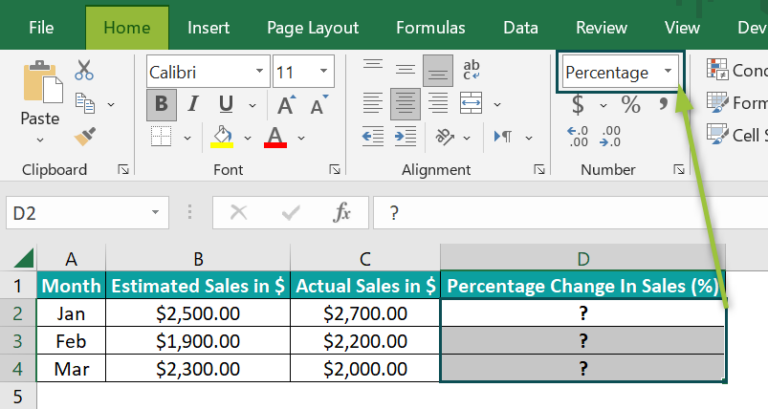The requirement is to determine the percentage change in sales each month and display the result in cell range D2:D4. Assume the data format of the target cells is Percentage.

Then, here is how to create the percentage change expression in Excel VBA to apply it in the target cells and get the required data.

• Step 1: Open the active worksheet containing the above table and press Alt + F11 to access the VBA Editor.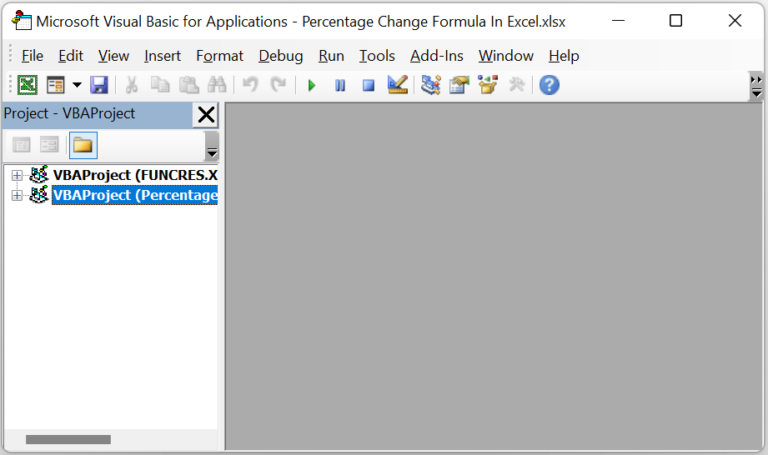• Step 2: Pick the required VBAProject and choose the Module option under the Insert tab to access a new module window.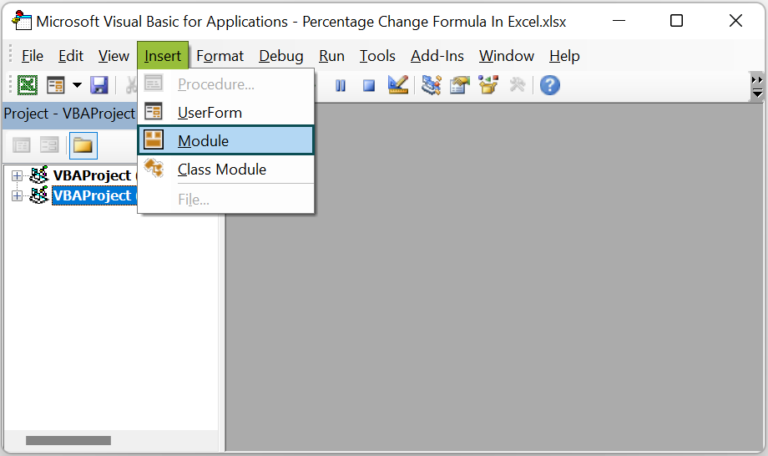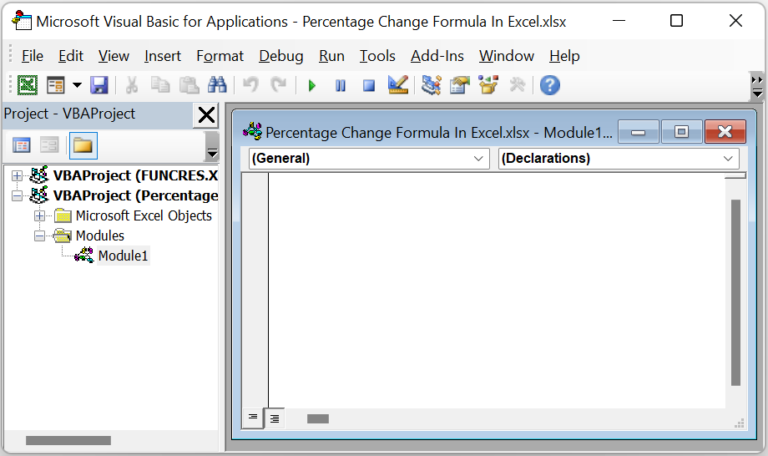• Step 3: Type the VBA code in the module window to apply the percentage change formula in the target cells.

Sub PercentChange_fn()
Dim ws As Worksheet
Set ws = Worksheets(“FAQ_2”)
ws.Range(“D2”) = (ws.Range(“C2”) – ws.Range(“B2”)) / ws.Range(“B2”)
ws.Range(“D3”) = (ws.Range(“C3”) – ws.Range(“B3”)) / ws.Range(“B3”)
ws.Range(“D4”) = (ws.Range(“C4”) – ws.Range(“B4”)) / ws.Range(“B4”)
End Sub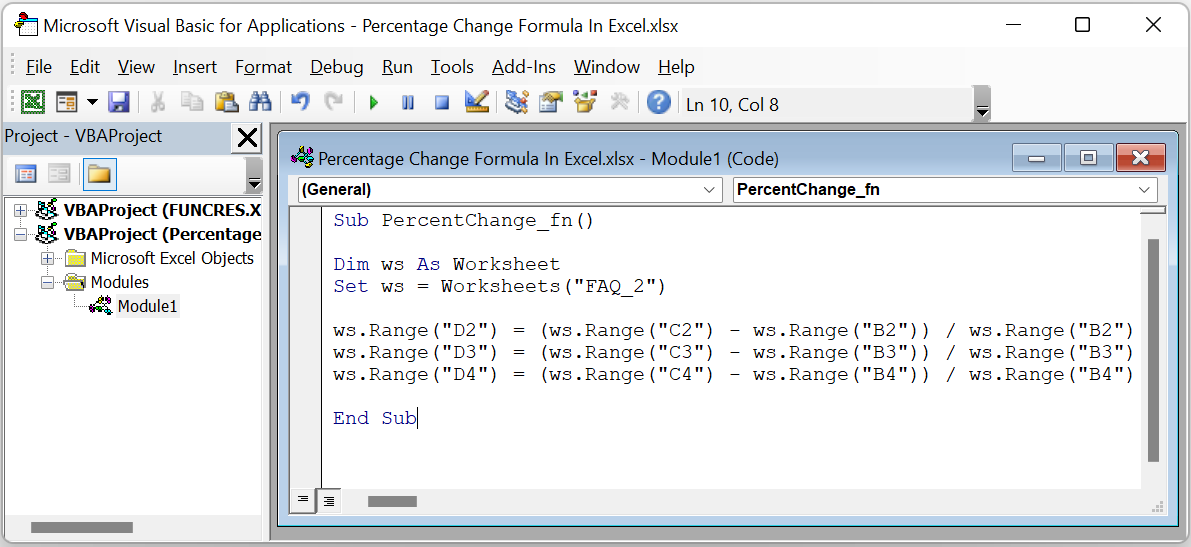• Step 4: Click the Run Sub/UserForm button to execute the code.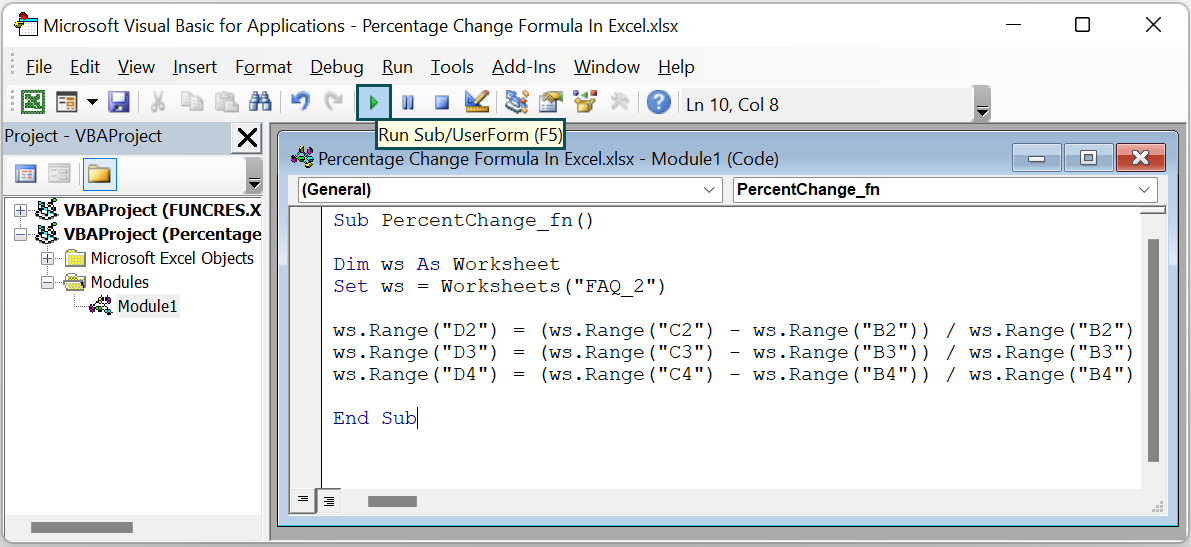We can now open the active worksheet to see the required monthly percent change values.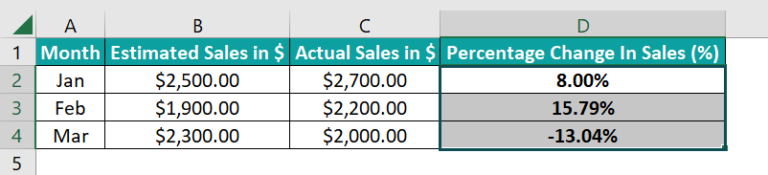3. What is the average percentage change formula in Excel?

The average percentage change formula in Excel is as follows:
Average Percentage Change = Sum of percentage Changes / Total Intervals

Where the formula for the percentage change is:
Percentage Change = ((Final_Value – Initial_Value) / Initial_Value) * 100

And the total intervals are the total number of percentage change values.